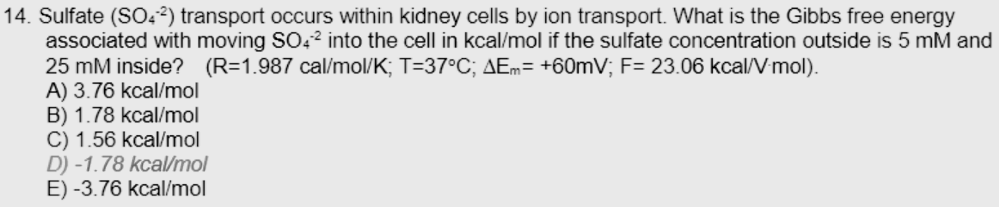# Problem: Sulfate (SO42-) transport occurs within kidney cells by ion transport. What is the Gibbs free energy associated with moving SO42- into the cell (in kcal/mol) if the sulfate concentration outside is 5mM and 25mM inside? (R = 1.987 cal/mol K; T=37ºC; ΔEm = +60mV; F = 23.06 kcal/V mol)A. 3.76 kcal/molB. 1.78 kcal/molC. 1.56 kcal/molD. -1.78 kcal/molE. -3.76 kcal/mol

###### FREE Expert Solution

Substance transport across a membrane creates a concentration gradient and particle movement from high concentration to lower concentration is expected following the second law of Thermodynamics. The Gibbs free energy can be computed following ΔG = RT ln(cin/cout). However, for charged species, additional free energy is generated due to the repulsion of like charges.###### Problem Details

Sulfate (SO42-) transport occurs within kidney cells by ion transport. What is the Gibbs free energy associated with moving SO42- into the cell (in kcal/mol) if the sulfate concentration outside is 5mM and 25mM inside?

(R = 1.987 cal/mol K; T=37ºC; ΔEm = +60mV; F = 23.06 kcal/V mol)

A. 3.76 kcal/mol

B. 1.78 kcal/mol

C. 1.56 kcal/mol

D. -1.78 kcal/mol

E. -3.76 kcal/mol